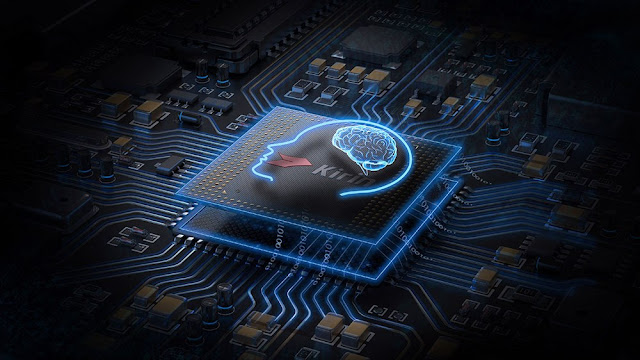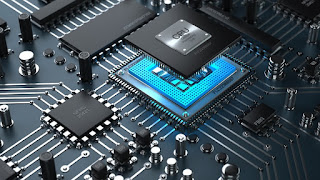# How the computers process Binary datas

## How the computer process binary data and how the bits work in the operating system?

The computer process binary data and produced output via PU

### Processing Unit(PU)

the computer has a processing unit to process that unit into 0 and 1. and produced output.Processing Unit

#### We have three types of processing unit in our system.GPU, CPU, and SPU
1. GPU= Graphic Processing System.
2. SPU= Sound Processing System.
3. CPU= Core Processing System.

So we used 0 and 1 for producing output data in pixels, it sounds, and in a mathematical process.

So depending on where is once and zero goes they produced output in a different type.graphics processing unit
GPU= Graphic Processing Unit

So we have a graphics processing unit. if we send our units and our bits over to the GPU and it processes our unit and produced the pixels in output.sound processing unit

#### SPU= Sound Processing Unit

If we sand our same units to the SPU and it processes our unit and produced the sound waves and send the signal to the speaker and produced the sound.core processing unit

#### CPU= Core Processing Unit

it's one of the more notable units. CPU is considered as a brain. it deals with logic so if we want to say to process the number like a calculator. our CPU is absolute is vital. because our CPU is performed complex calculations. and our programs are based on logic and logic should go to the core processing unit or the CPU. So it is dependent on the programmer to send the units to the different types of units

please comment on the section given below for any question